Select Page

# Manometers – Measurement of Pressure

## Get Quick and Accurate Online Homework Help from Qualified Tutors.#### 4.2 out of 5#### 4.7 out of 5#### 4.8 out of 5

• NHonest Pricing
• NMoney-back Guarantee

## Get Homework Help From ExpertsFREE QUOTE

Get a response in under 15 minIn the previous chapter, we have studied the pressure exerted by fluid on the wall of a container is calculated by using mathematical formula. In this chapter, we learn how to measure pressure using manometers and other mechanical gauges.

Manometers

Several instruments are used to measure pressure. A manometer is a device is used to measure pressure of a liquid by using the column of liquid. This column of liquid can be measured in vertical or inclined tubes. These devices are used for measuring different types of pressures that include absolute pressure, gauge pressure, atmospheric pressure and differential pressure.

There are different types of manometers available to measure these types of pressure. But manometers are mainly classified into two types:

1. Simple Manometer
2. Differential Manometer
1. Simple Manometer

Simple manometers are just tubes with open top. These are attached to the vessel at its top and used to measure pressure as compare to atmospheric pressure. Simple manometers are of different types. It can be classified into:

• Piezometer:

Piezometer is one of the simplest manometers with open top which is attached to a vessel containing liquid. The pressure is exerted by the liquid increases the level of liquid in the tube as shown in Fig. 3.1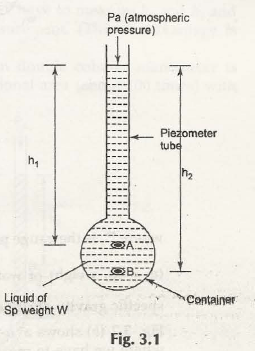Let us consider the height of point A from the upper surface of liquid in the piezometer be h1

Height of point B from the upper surface of liquid in the piezometer be h2

Pa be the atmospheric pressure

The fluid pressure at point A can be given by,

PA = h1w + Pa

The fluid pressure at point B can be given by,

PB = h2w + Pa

When both these pressures at point A and B need to be expressed in terms of gauge pressure, the term Pa will be zero. Hence, we get-

PA = h1w

PB = h2w

• U-tube Manometer:

The name suggests that it is of U-shape and the raise of liquid in both the tube is considered to measure the fluid pressure as shown in Fig. 3.2 (a) Double Column Manometer (Px> Pa)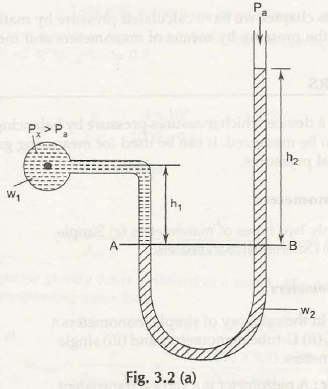Let us consider the gauge pressure in the container be Px

Pa be the atmospheric pressure exerts on the liquid in the U-tube manometer

w1be the specific gravity of liquid in the container

w2be the specific gravity of liquid in the U-tube manometer

h1 be the height of liquid in the container up to the datum line AB

h2 be the height of liquid in the U-tube manometer up to the datum line AB

So,

The pressure exerted by liquid at A is given by,

PA = Px + w1h1

Similarly, pressure exerted by liquid at B is given by

PB = Pa+ w2h2

Or,

PB = w2h2                                              (Pa= 0)

We know that,

PA = PB

Px + w1h1 = w2h2

Or,

Px = (w2h2 – w1h1)

If the head of water column is considered, then the specific gravity can be given by-

Px/ w = (w2h2 – w1h1)/ w                                  (w = specific weight of water)

Or,

Px/ w = (S2h2 – S1h1)

Here,

S1 and S2 = Specific Gravity of both liquids

Now,

Considering Fig. 3.2 (b) Double Column Manometer (Px< Pa)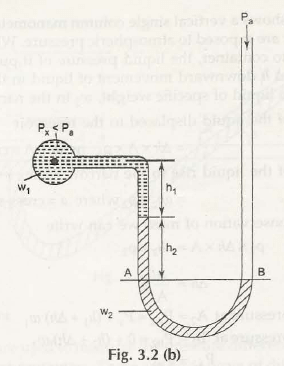The pressure exerted by liquid at A is given by,

PA = Px + w1h1 + w2h2

Similarly, pressure exerted by liquid at B is given by

PB = 0

We know that,

PA = PB

Px + w1h1 + w2h2 = 0

Or,

Px = – (w1h1+w2h2)

If the head of water column is considered, then the specific gravity can be given by-

Px/ w = – (w1h1+w2h2)/ w                                 (w = specific weight of water)

Or,

Px/ w = – (S1h1+S2h2)

Here,

S1 and S2 = Specific Gravity of both liquids

• Single Column Manometer:

Single Column Manometer is just like u-tube manometer whose one end is replaced with a reservoir of large cross-sectional area as compare to other limb as shown in Fig. 3.3 (a)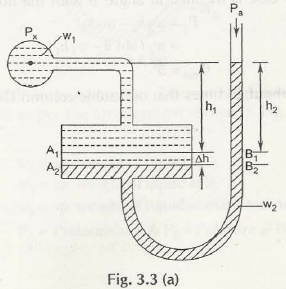Let us consider both the limbs of manometer are exposed to atmospheric pressure, Pa

When the limb (left one) with reservoir is connected to the container, the pressure of liquid exerts on it to push it down from A1B1 to A2B2.

The change in the level of liquid be h

h1 be the height of liquid in the container up to the line A1B1

h2 be the height of liquid in the manometer up to the line A1B1

w1be the specific gravity of liquid in the container

w2be the specific gravity of liquid in the manometer

So,

Mass of liquid displaced by liquid is given by,

m = h x A x Px

Similarly, Mass of liquid rise in the right limb is given by-

m = h2 x a x Px

According to law of conservation of masses, it can be written as-

h x A x Px = h2 x a x Px

Or,

h = h2 x a/ A

Now,

Gauge pressure at A2 is given by,

PA2 = Px + (h1 + h) w1

Similarly,

Gauge pressure at B2 is given by,

Pa2 = Pa + (h2 + h) w2

We know that,

PA2 = Pa2

Px + (h1 + h) w1 = Pa + (h2 + h) w2                           (Pa = 0)

Px = (w2h2 – w1h1) + h(w2 – w1)

Or,

Px = w2h2 (1 + a/ A) – w1 (h1 + h2.a/ A)

a/A is negligible as its value is nearly zero

Hence,

Px = (w2h2 – w1h1)

Considering the Fig. 3.3 (b)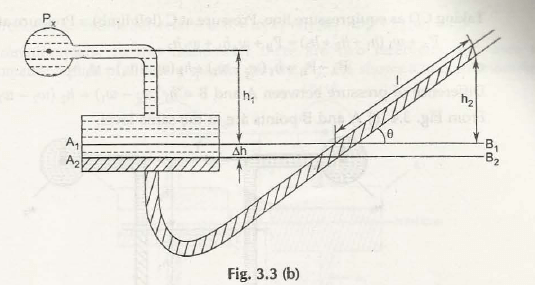It shows an inclined manometer with an angle  with horizontal then,

We know that,

Px = (w2h2 – w1h1)

= w2 sin – w1h1

Note:

Inclined manometer is considered as 25 times more sensitive than double column U-tube manometer.

1. Differential Manometers

Differential manometers measure the pressure difference between two different points in two different containers. It can be classified into three differential manometers:

• Differential U-tube manometer:

Differential U-tube manometer is similar to U-tube manometer and is used to measure pressure difference directly as shown in Fig. 3.4 (a)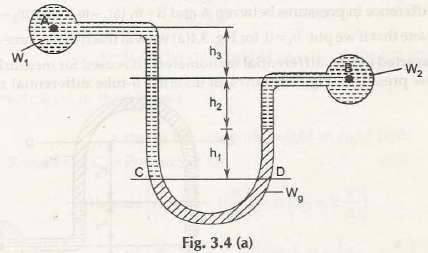Let us consider heavy liquid say mercury is present in the U-tube and it is attached to the ends of two different reservoirs.(Container A and B are placed at different heights)

w1be the specific gravity of liquid in container A

w2be the specific gravity of liquid in container B

wgbe the specific gravity of mercury in U-tube differential manometer

h1 be the height of mercury from the CD in U-tube differential manometer

h2 be the height of liquid in the container B above the mercury level

h3 be the height of liquid in the container Aup to the level of h2

We know that,

Pressure at A, PA = Pressure at B, PB

Similarly,

Pressure at C, PC = Pressure at D, PD                                      (For Equipressure line)

PA + w1(h1 + h2+ h3) = PB+ wgh1+w2h2

PA –PB = wgh1+w2h2 – w1(h1 + h2 + h3)

= h1(wg– w1) + h2(w2– w1) – w1h3

This is the pressure difference between A and B

Suppose container A and B are in the same level as shown in Fig. 3.4 (b)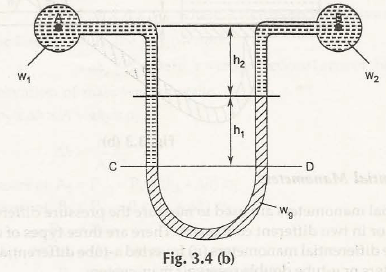Here,

h1 be the height of mercury from the CD in U-tube differential manometer

h2 be the height of liquid in the above the mercury level

Now,

Pressure at C, PC = Pressure at D, PD                                      (For Equipressure line)

PA + w1(h1 + h2) = PB + wgh1+w2h2

PA –PB= wgh1+w2h2 – w1(h1 + h2)

= h1(wg – w1) + h2 (w2 – w1)

Note:

If we put the value of h3 = 0, then same equation can be achieved from the above equation for Fig. 3.4 (a).

• Inverted U-tube differential manometer

Inverted U-tube differential manometer is used to measure low pressure difference as shown in Fig. 3.5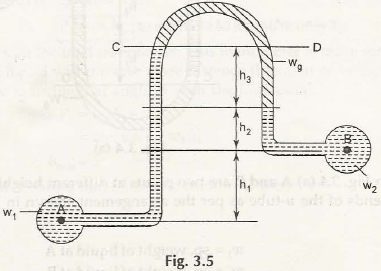Let us consider heavy liquid say mercury is present in the U-tube and it is attached to the ends of two different reservoirs similar to U-tube differential manometer. (Container A and B are placed at different heights)

w1be the specific gravity of liquid in container A

w2be the specific gravity of liquid in container B

wgbe the specific gravity of mercury in inverted U-tube differential manometer

h1 be the height of liquid in the container A up to the level of h2

h2 be the height of liquid in the container B below the mercury level

h3 be the height of mercury from the CD in inverted U-tube differential manometer

We know that,

Pressure at C, PC = Pressure at D, PD                                      (For Equipressure line)

PA – w1(h1 + h2 + h3) = PB– wgh1–w2h2

PA –PB= w1(h1 + h2 + h3)– wgh1–w2h2

= h3(w1– wg) + h2 (w1– w2) – w1h1

This is the pressure difference between A and B

• Micro manometer:

This type of manometers consists of two reservoirs having large cross-sections and contains two different liquids of different specific gravity as shown in Fig. 3.6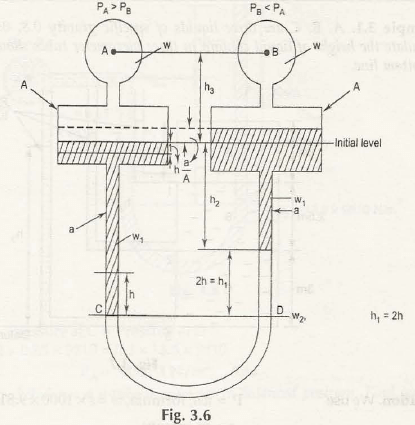Let us consider standard liquid is present in the tube and it is attached to the ends of two different reservoirs. (Reservoir A and B are placed at same heights)

w1 be the specific gravity of liquid in reservoir A

w2 be the specific gravity of liquid in reservoir B

w be the specific gravity of standard liquid in the tube

h be the height of liquid (fall) in the reservoir A

h1 be the height of standard liquid (rise) in the reservoir B

h2 be the height of liquid in the reservoir B up to the initial level mark

h3 be the height of line AB up to the initial level mark

Now,

Fall of standard liquid in reservoir A = Rise of standard liquid in reservoir B = h. a/ A

We know that,

Pressure at C, PC = Pressure at D, PD                                      (For Equipressure line)

PA+ w1(h1 + h2– h. a/ A) + w (h3 +h. a/ A) = PB+ w1 (h2 +h. a/ A) + w2h1+w (h3 –h. a/ A)

Consider h. a/ A is negligible as its value is nearly 0, then-

PA + w1(h1 + h2) + w (h3) = PB + w1 (h2) + w2h1+w (h3)

PA –PB = w1h2 + w2h1+wh3 – w1(h1 + h2)– wh3

= h1(w2– w1)

Or,

PA –PB = 2h(w2 – w1)                            (h1 = 2h)

This is the pressure difference between A and B

Some Basic Problems on Manometers

Example 3.1:

Consider the Fig. 3.7 to calculate height of liquid column in three different piezometer tubes.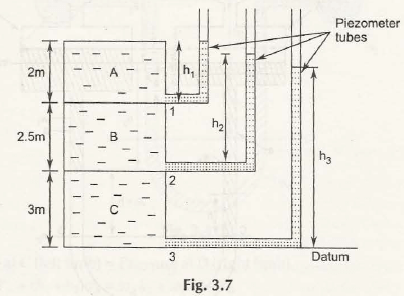Take specific gravity of three liquids as 0.8 for A, 0.85 for B and 0.95 for C.

Solution:

We know that,

Pressure, P = w x h                                          (w = S x 1000 x 9.81 N/m3)

Where,

S = Specific Gravity of liquid

Pressure at point 1:

P1 = 0.8 x 1000 x 9.81 x 2

= 15696 N/m2

Thus,

Height of liquid column will be given by,

h1 = 15696/ (0.8 x 1000 x 9.81)

= 2 m

Pressure at point 2:

P2 = (0.85 x 1000 x 9.81 x 2.5) + (0.8 x 1000 x 9.81 x 2)

= 36542 N/m2

Thus,

Height of liquid column will be given by,

h2= 36542/ (0.85 x 1000 x 9.81)

= 4.4 m

Pressure at point 3:

P3 = (0.95 x 1000 x 9.81 x 3) + (0.85 x 1000 x 9.81 x 2.5) + (0.8 x 1000 x 9.81 x 2)

= 64501 N/m2

Thus,

Height of liquid column,h3= 64501/ (0.95 x 1000 x 9.81)

= 6.92 m

Example 3.2:

A U-tube manometer is shown in Fig. 3.8. Find the vacuum pressure at the point A.

Solution:

From the given data, the equation for finding pressure at point A can be written as-

PA + 0.2 x 0.9 x 9810 + 0.5 x 13.6 x 9810 = 0

Hence,

PA = – 98474 N/m2

Links of Next Mechanical Engineering Topics:-

## Benefits of Online Homework Help### 70+ Subjects

Our team of professional academic writers are experts in their fields and are well-equipped to handle any complex subject. You can always count on one of our specialists to assist you.We understand the importance of meeting deadlines and we pride ourselves on being able to deliver tasks quickly. No matter how tight the deadline, we will complete your homework on time.### Chat with Expert

Stay in touch with your expert by utilizing our secure chatboard for all your queries and concerns. You can easily check progress and make sure your work is on the right track.### Free Revisions

We strive to ensure complete customer satisfaction. We offer free revisions to make sure our clients are happy with the final product. Your satisfaction is our top priority.

## Reviews from Customers

4.5
Rated 4.5 out of 5
4.5 out of 5 stars (based on 466 reviews)
Excellent58%
Very good34%
Average8%
Poor0%
Terrible0%

C
E

#### How do I submit my assignment?

Getting assignment help is very simple with us. Students can either send us the homework via email or they can upload it to our online form here. For a quicker response, You can also chat with us at WhatsApp and submit homework directly. You are sure to get a response from our side within 10 minutes. You can pay for your order using secure PayPal or major credit card gateways.

C
E

#### Who will do my homework?

All our tutor experts are highly qualified professionals – holding at least Master’s degree in their fields. Currently, our tutor pool is made of 400 subject-specific experts.

Each expert has been individually chosen after a series of rigorous screen tests, which involves credential checks, work history analysis, writing sample assessment, and one-on-one interviews.

C
E

#### How much will my homework cost?

Each assignment is quoted a unique price. It is based on (i) complexity of task, (ii) your deadline and (iii) tutor’s estimate of the time it will take them to complete your homework. There are no obligations, and you are free to discuss the price quote with the tutor.

We also accept partial payment to start working on your assignment help. You can pay the remaining amount when your task gets completed. No pressure of up-front payment. No hidden order costs. We do not have a monthly fees or minimum payments for services.

C
E

#### Can I pay someone to help me with my homework?

Yes, you can pay online tutors for homework help. There is nothing wrong with asking for help from trained and professional subject tutors to clarify doubts regarding any assignment or coursework.

C
E

#### What mode of payment is acceptable?

There are several ways to pay for homework help:

• Credit/Debit Card: You can use your credit or debit card to make a payment online. We accept VISA/MASTERCARD/AMEX.
• PayPal is a secure online payment service that allows you to pay for essay writing services using your account balance or linked bank account.

C
E

#### Can I chat with my tutor?

Using our secure chat board, you can now chat directly with your assigned tutor. The chats are encrypted both ways to secure your privacy. This makes your contact with the tutor directly & confidentially, so you can better explain any requirements or changes if needed or just need updates.

You can't contact the experts outside of chat board platform. Sharing any personal information, including but not limited to contact information, goes against our Terms and Conditions and therefore may result in permanently blocking you from the platform. We take any personal data very seriously and we do it for the safety of our users.

C
E

#### Can I get free online homework help?

Our homework services are not free because we provide customized, high-quality homework solutions. We have a prepaid system that ensures professional homework helpers are paid fairly for their work and time. We believe that the value of their expertise and the effort they put into crafting each assignment justifies the cost of our service.
C
E

#### Is My Homework Help Legit?

My Homework help is absolutely legit! We are the leading providers of college homework services since 2012. We connect you with experienced tutors for your assignment and make sure you get completed answers before deadline. Our guarantee is high-quality assignment solutions or your money-back. It’s that risk-free! Furthermore, everything about you is kept confidential.

C
E

#### Can I trust you?

My Homework Help is absolutely reliable. Students of the world's top universities use our services and 50% of our customers are repeat customers. Our ordering process is very simple & transparent. There are no hidden fees or subscription anything like that. You can also request a refund if you think that our expert wasn’t able to meet your requirements. Your prime satisfaction is our end goal!

Know more - How it works

C
E

#### What about privacy & confidentiality?

Using My Homework Help is absolutely safe. We care about your security, therefore we encrypt all personal data to make every user feel safe while using our services and we don’t share any personal information with any third parties without your permission. Your credit card information is not stored anywhere at My Homework Help, and use of PayPal relies on their secure payment networks. Your identity, payment and homework are in safe hands. You can always be certain of getting professional help and remaining anonymous, while using My Homework Help.